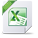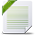# Power BI User Group of Philadelphia

View Only

## Having Fun with DAX and Power BI: Creating a Summarized Table and Appending a Total Row (Without Power Query)

• #### 1.  Having Fun with DAX and Power BI: Creating a Summarized Table and Appending a Total Row (Without Power Query)

Posted Jul 11, 2020 09:38 PM

Having Fun with DAX and Power BI: Creating a Summarized Table and Appending a Total Row (Without Power Query)

Of course, there are many considerations that must be accounted for and that this may not be the best way to accomplish aggregating a table and appending a total row (nor why would you need or want to).  However, this article is about how to accomplish cool tricks with Power BI and the Powerfulness of DAX and that you may find a use case to do so.

Let us first start off with a simple table of data to be aggregated, that looks like this (data file provided via excel and created using mockaroo.com):Now using the table, you could create some measures and create some charts. Here are a few examples:

• _Total Initial Trainings = COUNTA('Program'[DateofFirstTraining])
• _Total Additional Trainings = CALCULATE(SUM('Program'[Trainings])) - [_Total Initial Trainings]
• _Total Trainings = [_Total Additional Trainings] + [_Total Initial Trainings]Now, we can summarize the same information to create an AgeGroup Table such as

• Age Group Table 1 = SUMMARIZE('Program','Program'[AgeGroup],"Initial Trainings",[_Total Initial Trainings],"Additional Trainings",[_Total Additional Trainings]))

That would result in the following table and the same chartTo get a total row to add to your visual, we will get a little creative and rewrite the code using the GENERATE, ROW, and UNION functions.We begin by rewriting this table code

As the following:

• VAR ageTotals = GENERATE(SUMMARIZE('Program','Program'[AgeGroup]) ,ROW ( "Initial Trainings" ,

VAR ageID = Program[AgeGroup]

RETURN CALCULATE( [_Total Initial Trainings],FILTER('Program' , 'Program'[AgeGroup] = ageID) , 'Program'[DateofFirstTraining]),"Total Trainings" ,

VAR ageID = Program[AgeGroup]

FILTER('Program' , 'Program'[AgeGroup] = ageID)) ))

RETURN ageTotals

This code generates the same summarized table and stores it into a table variable that is then returned.  We will change the RETURN Statement later. As we continue building on this table, we create a new variable that aggregates all the values as a row.

• VAR AggregatedTotal =   GENERATE(SUMMARIZE('Program'," ",SWITCH(TRUE(),SUM('Program'[Trainings])>0,"Total")),

ROW("Initial Trainings", SUMX(

FILTER(ALL('Program') , 1=1 ) , [_Total Initial Trainings]          ) ,

If we return the AggregatedTotal variable, we get a table as follows:Now the key is to get the Total added to the table is to union the two variables as one table.  That is where the UNION function is used in the RETURN statement as:

• RETURN UNION(ageTotals,AggregatedTotal)

which gives you the following tableWhich now I can create the following chart------------------------------
Brian Connelly